# 1. Given force F-xi 4zj Path 1: (0,0,0) to (0,1,1) Path 2: (0,1,1) to (1,1,0) Path 3: (1,1,0) to (0,0,0) 5yk acts follo...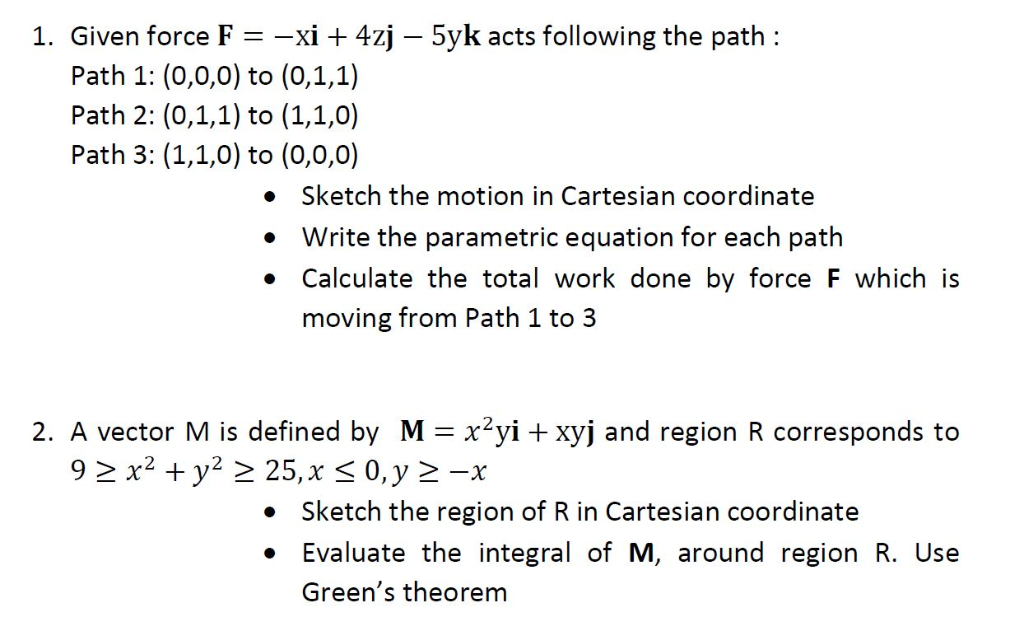1. Given force F-xi 4zj Path 1: (0,0,0) to (0,1,1) Path 2: (0,1,1) to (1,1,0) Path 3: (1,1,0) to (0,0,0) 5yk acts following the path: . Sketch the motion in Cartesian coordinate Write the parametric equation for each path Calculate the total work done by force F which is moving from Path 1 to 3 2. A vector M is defined by M-x2yi xyj and region R corresponds to Sketch the region of R in Cartesian coordinate . Evaluate the integral of M, around region R. Use Green's theorem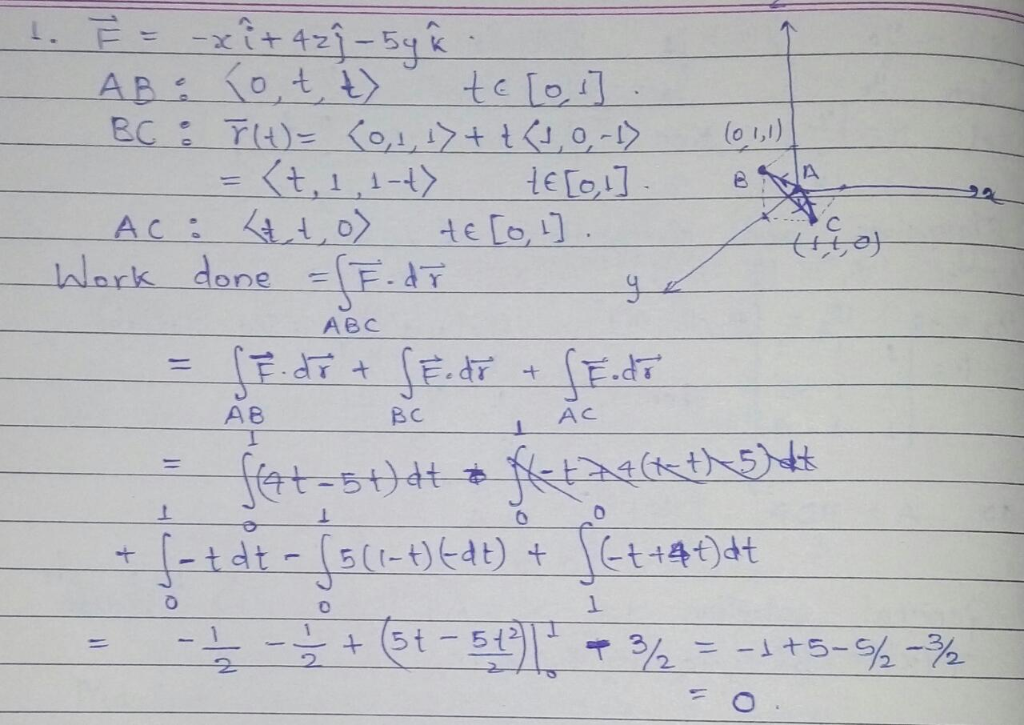first complete question according to chegg policy.

##### Add Answer of: 1. Given force F-xi 4zj Path 1: (0,0,0) to (0,1,1) Path 2: (0,1,1) to (1,1,0) Path 3: (1,1,0) to (0,0,0) 5yk acts follo...
Similar Homework Help Questions
• ### Assignment # 2 (40 Points] (Page 1/2) A force of magnitude F is on the r-y...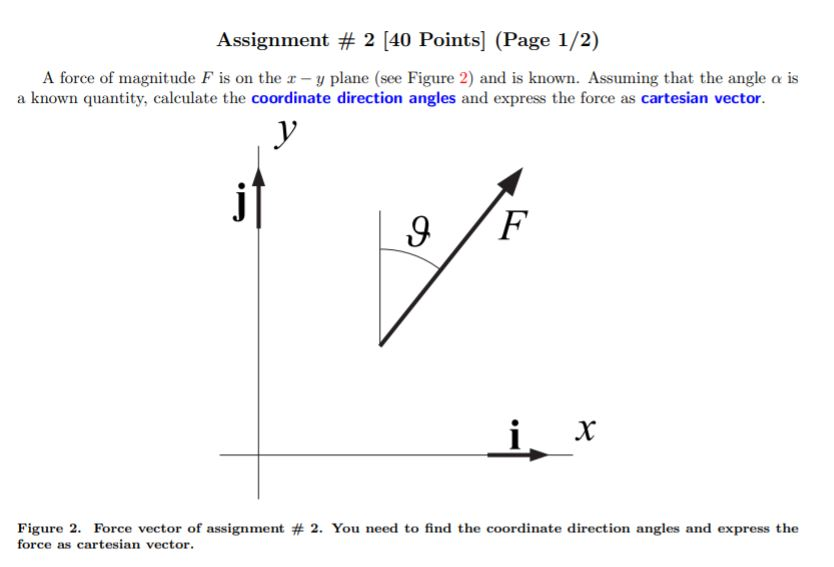Assignment # 2 (40 Points] (Page 1/2) A force of magnitude F is on the r-y plane (see Figure 2) and is known. Assuming that the angle a is a known quantity, calculate the coordinate direction angles and express the force as cartesian vector F Figure 2. Force vector of assignment # 2. You need to find the coordinate direction angles and express the force as cartesian vector.

• ### solve clearly please 2. Consider the curve C and the vector F in 1 again. (1) Calculate the line integral in (3) of 1 by using Green's Theorem (P,Q, R), Jc F dr-Sc Pdz+Qdy + Rd Hint: For...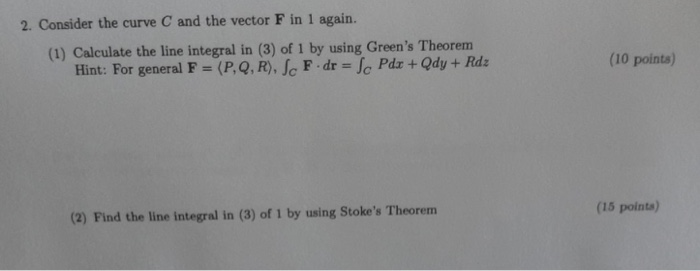solve clearly please 2. Consider the curve C and the vector F in 1 again. (1) Calculate the line integral in (3) of 1 by using Green's Theorem (P,Q, R), Jc F dr-Sc Pdz+Qdy + Rd Hint: For general F (10 points) (2) Find the line integral in (3) of 1 by using Stoke's Theorem (15 points) t 5-l on ry-plane can be expressed as C: r(9) i. Curve (208θ, 3sine,o), o S 2r. Let F (v,z, 2) be a...

• ### 1) Force F =(-8.00 N){+(6.00 N) j acts on a particle with position vector r =...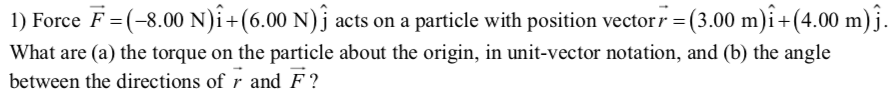1) Force F =(-8.00 N){+(6.00 N) j acts on a particle with position vector r = (3.00 m)i +(4.00 m)j. What are (a) the torque on the particle about the origin, in unit-vector notation, and (b) the angle between the directions of r and F?

• ### Please help! Question 5 25 (5.1) Sketch some vectors in the vector field given by F(r,...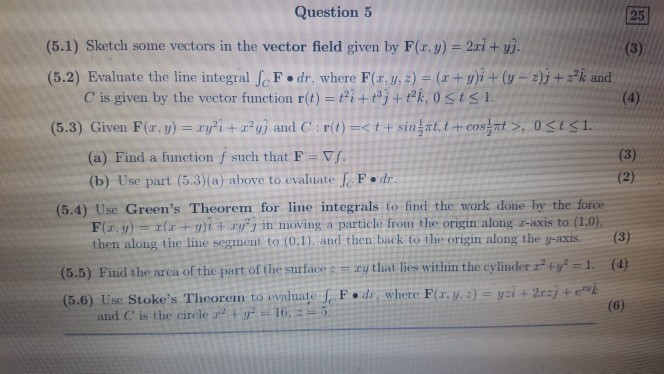Please help! Question 5 25 (5.1) Sketch some vectors in the vector field given by F(r, y) 2ri + yj. (3) (5.2) Evaluate the line integral fe F dr, where F(r, y, 2) = (x + y)i + (y- 2)j+22k and C is given by the vector function r(t) = ti + #j+Pk, 0 <t<1 (4) costrt>, 0St<1 (5.3) Given F(r, y) = ryi + yj and C: r(t)=< t + singat, t (3) (a) Find a function f such...

• ### solve clearly please 1. Curve1 on zy-plane can be expressed as C:r(0) - (2cos0, 3sin 0,0), 0 s e s 2r. Let F (v,z, z) be a given vector. (1) Find the line integral Jo zdo (5 points) (2) Find the...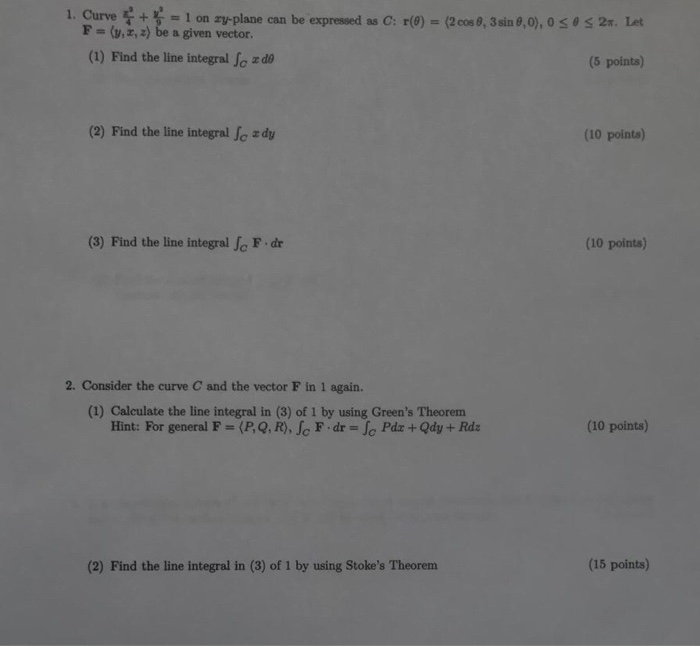solve clearly please 1. Curve1 on zy-plane can be expressed as C:r(0) - (2cos0, 3sin 0,0), 0 s e s 2r. Let F (v,z, z) be a given vector. (1) Find the line integral Jo zdo (5 points) (2) Find the line integral Jo ady (10 points) (3) Find the line integral Jo F dr (10 points) 2. Consider the curve C and the vector F in 1 again. (1) Calculate the line integral in (3) of 1 by using...

• ### (2 pts) Calculate the circulation, rF dr, in two ways, directly and using Stokes' Theorem. The vector field F (...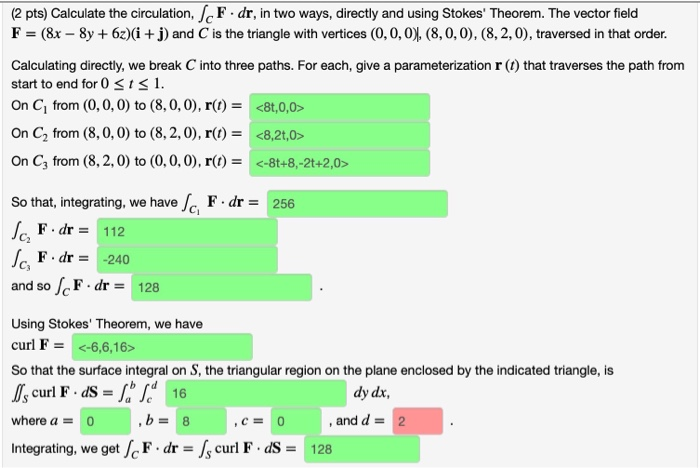(2 pts) Calculate the circulation, rF dr, in two ways, directly and using Stokes' Theorem. The vector field F (8x-8y+62)(i + j) and C is the triangle with vertices (0,0,0), (8, 0, 0), (8,2,0), traversed in that order. Calculating directly, we break C into three paths. For each, give a parameterization r (t) that traverses the path from start to end for 0sts 1 On Ci from (0,0, 0) to (8,0,0), r(t) = <8t,0,0> On C2 from (8, 0, 0)...

• ### The force field F (x, y) = (x + 4y)i + (x^2 − 3)j acts on an object traveling from (0, 0) to (0, 1). The object moves along the path x = c(y − y^2 ) with 0 ≤ y ≤ 1. Determine the value of c that minim...

The force field F (x, y) = (x + 4y)i + (x^2 − 3)j acts on an object traveling from (0, 0) to (0, 1). The object moves along the path x = c(y − y^2 ) with 0 ≤ y ≤ 1. Determine the value of c that minimizes the work done on the object by the force field. Please use the line work integral, and optimization

• ### sin2 0+cos2 0=1 sin2 0=1-cos 20 cos2 0+cos 20 cos 20 cos2 0- sin2 sin 20...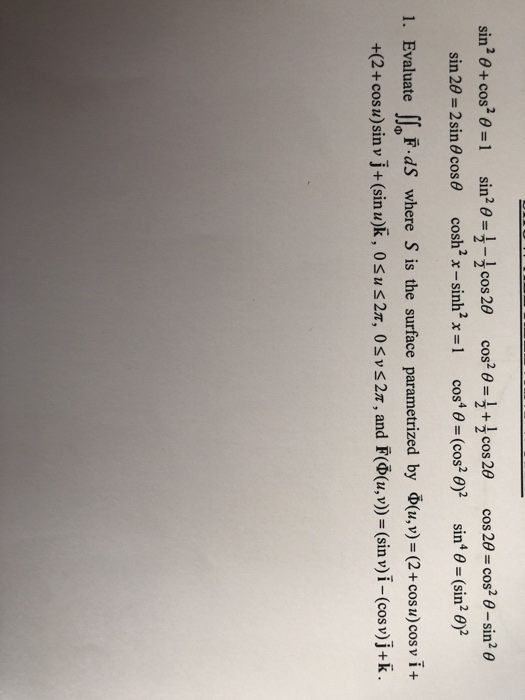sin2 0+cos2 0=1 sin2 0=1-cos 20 cos2 0+cos 20 cos 20 cos2 0- sin2 sin 20 2 sin 60 cos cosh2 x-sinh2 x =1 cos4 0 (cos2 0)2 sin 0= (sin2 0)2 1. Evaluate F dS where S is the surface parametrized by (u,v)= (2+ cos u) cos v i + +(2+ cosu)sin v j+(sin u)k, 0su 27, 0svs 27, and F((u,v))= (sin v)i -(cos v)j+k 2. Verify Green's Theorem for the function F(x, y) = (2y+3) i+(-5x)j where D...

• ### All of 10 questions, please. 1. Find and classify all the critical points of the function. f(x,y) - x2(y - 2) - y2 » 2. Evaluate the integral. 3. Determine the volume of the solid that is inside the...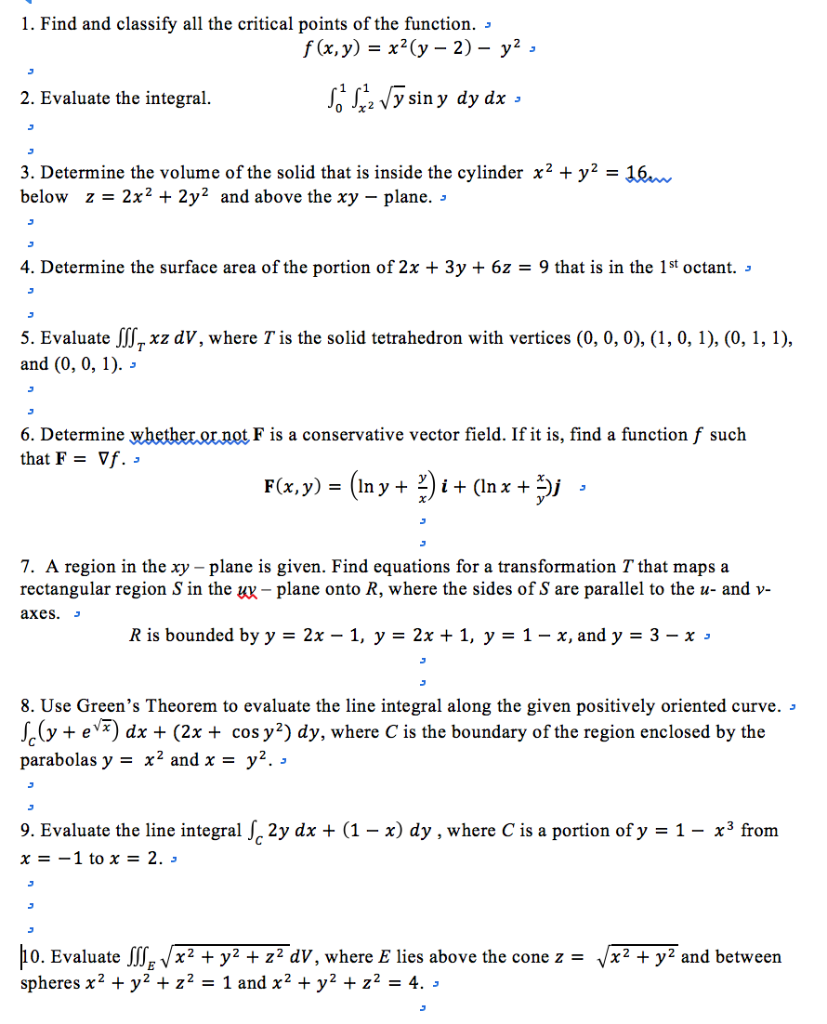All of 10 questions, please. 1. Find and classify all the critical points of the function. f(x,y) - x2(y - 2) - y2 » 2. Evaluate the integral. 3. Determine the volume of the solid that is inside the cylinder x2 + y2- 16 below z-2x2 + 2y2 and above the xy - plane. 4. Determine the surface area of the portion of 2x + 3y + 6z - 9 that is in the 1st octant. » 5. Evaluate JSxz...

• ### 6. Let F : Rn → Rn, n 1, 2, 3, , be a conservative force....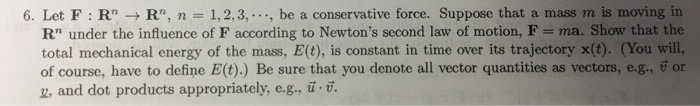6. Let F : Rn → Rn, n 1, 2, 3, , be a conservative force. Suppose that a mass m is moving in Rn under the influence of F according to Newton's second law of motion, F ma. Show that the total mechanical energy of the mass, E(t), is constant in time over its trajectory x(t). (You will, of course, have to define Et),) Be sure that you denote all vector quantities as vectors, e-g, or z, and dot...

Need Online Homework Help?